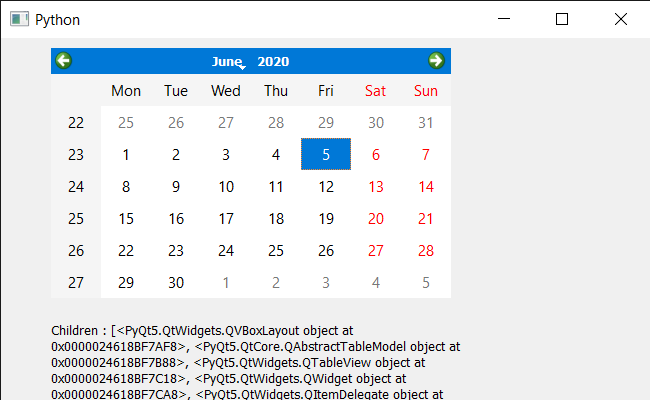# PyQt5 QCalendarWidget – Getting Children

• Last Updated : 02 Nov, 2021

In this article we will see how we get the children of the QCalendarWidget. Calendar is not alone a single widget it is a mixture of lots of smaller widget which we refer as calendar’s child. There are many child such as table view, item delegate etc.

In order to do this we will use children method with the QCalendarWidget object.
Syntax : calendar.childAt()
Argument : It takes no argument
Return : It return list of all the child object of calendar

Below is the implementation

## Python3

 `# importing libraries``from` `PyQt5.QtWidgets ``import` `*``from` `PyQt5 ``import` `QtCore, QtGui``from` `PyQt5.QtGui ``import` `*``from` `PyQt5.QtCore ``import` `*``import` `sys`  `class` `Window(QMainWindow):` `    ``def` `__init__(``self``):``        ``super``().__init__()` `        ``# setting title``        ``self``.setWindowTitle(``"Python "``)` `        ``# setting geometry``        ``self``.setGeometry(``100``, ``100``, ``650``, ``400``)` `        ``# calling method``        ``self``.UiComponents()` `        ``# showing all the widgets``        ``self``.show()` `    ``# method for components``    ``def` `UiComponents(``self``):` `        ``# creating a QCalendarWidget object``        ``self``.calendar ``=` `QCalendarWidget(``self``)` `        ``# setting geometry to the calendar``        ``self``.calendar.setGeometry(``50``, ``10``, ``400``, ``250``)` `        ``# creating a label``        ``label ``=` `QLabel(``self``)` `        ``# setting geometry``        ``label.setGeometry(``50``, ``280``, ``420``, ``120``)` `        ``# making it multi line``        ``label.setWordWrap(``True``)` `        ``# getting children``        ``value ``=` `self``.calendar.children()` `        ``# setting text to the label``        ``label.setText(``"Children : "` `+` `str``(value))`   `# create pyqt5 app``App ``=` `QApplication(sys.argv)` `# create the instance of our Window``window ``=` `Window()`  `# start the app``sys.exit(App.``exec``())`

Output :My Personal Notes arrow_drop_up